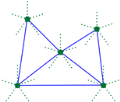CGAL 5.3 - Cone-Based Spanners
Cone-Based Spanners ReferenceWeisheng Si, Quincy Tse and Frédérik Paradis
This package provides functors for constructing two kinds of cone-based spanners: Yao graph and Theta graph, given a set of vertices on the plane and the directions of cone boundaries. Both exact and inexact constructions are supported. In exact construction, the cone boundaries are calculated using the roots of polynomials, thus avoiding the use of $$\pi$$, which cannot be represented exactly. In inexact construction, the cone boundaries are calculated using the approximate $$\pi$$ value defined in CGAL, which is still accurate enough for most applications. Moreover, for visualization purpose, this package provides a global function to generate the data and script files used by Gnuplot to plot the constructed graphs. This package also provides options for the Half Yao graph and the Half Theta graph.
Introduced in: CGAL 4.9
BibTeX: cgal:st-cbs-21b

## Functors

• CGAL::Compute_cone_boundaries_2
• CGAL::Construct_yao_graph_2
• CGAL::Construct_theta_graph_2

## Functions

• CGAL::gnuplot_output_2()

## Enumerations

• CGAL::Cones_selected

## Classes

class  CGAL::Compute_cone_boundaries_2< Traits_ >
The functor for computing the directions of cone boundaries with a given cone number and a given initial direction. More...

class  CGAL::Construct_theta_graph_2< Traits_, Graph_ >
A template functor for constructing Theta graphs with a given set of 2D points and a given initial direction for the cone boundaries. More...

class  CGAL::Construct_yao_graph_2< Traits_, Graph_ >
A template functor for constructing Yao graphs with a given set of 2D points and a given initial direction for the cone boundaries. More...

## Enumerations

enum  CGAL::Cones_selected { CGAL::EVEN_CONES = 0, CGAL::ODD_CONES = 1, CGAL::ALL_CONES = 2 }
An enum of the choice of cones in cone spanners. More...

## Functions

template<typename Graph >
void CGAL::gnuplot_output_2 (const Graph &g, const std::string &prefix)
outputs a set of files used by Gnuplot to plot g. More...

## ◆ Cones_selected

 enum CGAL::Cones_selected

#include <CGAL/Cone_spanners_enum_2.h>

An enum of the choice of cones in cone spanners.

Enumerator
EVEN_CONES

selects even cones.

ODD_CONES

selects odd cones.

ALL_CONES

selects all cones.

## ◆ gnuplot_output_2()

template<typename Graph >
 void CGAL::gnuplot_output_2 ( const Graph & g, const std::string & prefix )

#include <CGAL/gnuplot_output_2.h>

outputs a set of files used by Gnuplot to plot g.

The files that are generated for Gnuplot are: (1) prefix.v (vertex list) (2) prefix.plt (Gnuplot script), This script will read prefix.v as input to plot the vertex list. The edge list is also included in this script.

Notes: (1) If these files already exists, this function will overwrite these files. (2) Parallel and self-edges cannot be plotted.

Template Parameters
 Graph The type of the graph to be plotted. For this function to work, the graph type must be boost::adjacency_list with CGAL::Point_2 as the VertexProperties.
Parameters
 g A boost::adjacency_list graph with CGAL::Point_2 as the VertexProperties to be plotted prefix The prefix of the output files names
Examples:
Cone_spanners_2/theta_io.cpp.